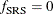Design Effect

If you specify the DEFF option in the MODEL statement, PROC SURVEYREG calculates the design effects for the regression coefficients. The design effect of an estimate is the ratio of the actual variance to the variance computed under the assumption of simple random sampling: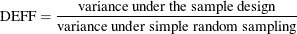See Kish (1965, p. 258) for more details. PROC SURVEYREG computes the numerator as described in the section Variance Estimation. And the denominator is computed under the assumption that the sample design is simple random sampling, with no stratification and no clustering.

To compute the variance under the assumption of simple random sampling, PROC SURVEYREG calculates the sampling rate as follows. If you specify both sampling weights and sampling rates (or population totals) for the analysis, then the sampling rate under simple random sampling is calculated as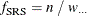where n is the sample size and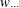(the sum of the weights over all observations) estimates the population size. If the sum of the weights is less than the sample size,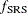is set to zero. If you specify sampling rates for the analysis but not sampling weights, then PROC SURVEYREG computes the sampling rate under simple random sampling as the average of the stratum sampling rates: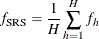If you do not specify sampling rates (or population totals) for the analysis, then the sampling rate under simple random sampling is assumed to be zero: